# Biomedical Engineering Logs & Exponents: Bode Plots

## Introduction

The Bode plot is an example of logarithmic graphing used by electrical engineers.

The voltage gain of a passive or active filter, as a function of frequency, is non-linear. For the high pass R-C filter, the magnitude of the voltage gain, as a function of frequency, is expressed by the formula: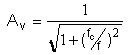where "fc" is called the cutoff frequency and "f" is the frequency in Hertz. "Av " is the voltage gain, (in this case, a loss) for the passive filter circuit.

Likewise, the graph of the voltage gain for a typical low-pass R-C filter is shown in the figure (1). A logarithmic scale is used to plot the frequency along the horizontal axis.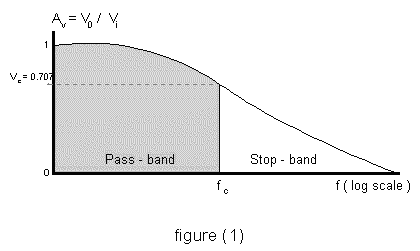For low frequencies, the gain of the circuit is equal to 1. This is called the pass-band region. The upper limit for the pass-band region is located at the cutoff frequency, "fc". The Bode db gain at the cutoff frequency is: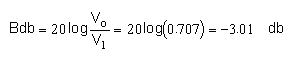## The Problem:

Given a high pass filter and R= 1.0 kΩ and C = 0.1 μF:

1. Calculate the cutoff frequency.
2. Calculate the Bode gain at the cutoff frequency.
3. Plot the Bode gain verses frequency, in Hertz, where the Bode gain is given by the formula: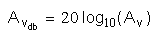4. Sketch the straight-line approximation or "idealized Bode plot".

## Solution:

1. The cutoff frequency for a high-pass R-C filter is: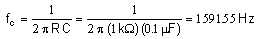2. The Bode gain at the cutoff frequency is: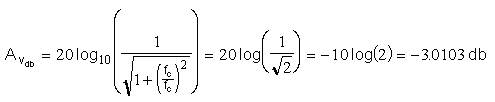3. The Bode plot versus frequency is shown below. Excel is used to generate the data.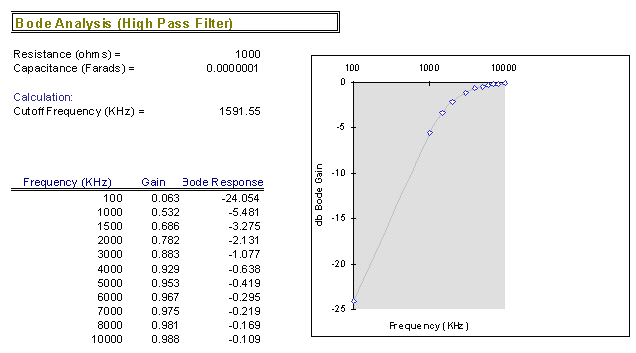4. The idealized Bode plot is obtained by considering the Bode gain: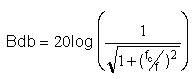The following approximations can be made:

Case (i):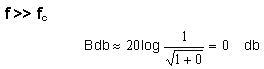The Bode gain in the pass-band region is zero.

Case (ii):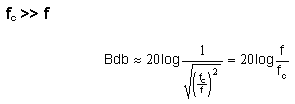For the stop-band region, the Bode gain is a straight line with slope equal to 20 db per decade. The Bode gain is negative, intersecting with the pass-band approximation at the cutoff frequency.